The full adder circuit is a fundamental building block of digital electronics and is essential for carrying out arithmetic operations in binary form. It is used to add two binary numbers and generate a sum output and a carry output.

The full adder is a more complex circuit compared to the half adder, which can only add two binary digits without taking into account any carry from the previous addition. The full adder circuit, on the other hand, takes into account the carry from the previous addition and produces the correct sum and carry outputs.

An adder is a digital circuit used in computers and other kinds of processors that performs addition of binary numbers by taking two input numbers and generating a sum output.

The full adder circuit is more complex than the half adder, as it takes into account the carry from the previous addition and produces the correct sum and carry outputs .

Adders can be constructed for many number representations, but most commonly operate on binary numbers. In cases where two’s complement or one’s complement is being used to represent negative numbers, it is trivial to modify an adder into an adder–subtractor. Other signed number representations require more logic around the basic adder.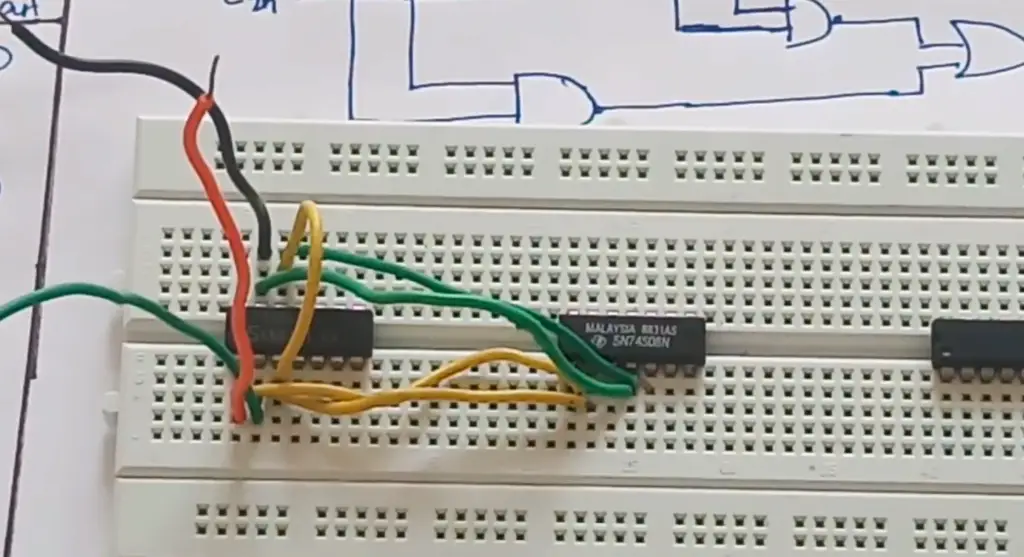## What is a Full Adder Circuit?

A Full Adder circuit is a digital logic circuit used for adding three binary bits and generating two outputs, which are the sum bit and carry bit.

It is a combinational logic circuit made up of multiple logic gates like AND, OR, and XOR gates. The Full Adder circuit is a building block for more complex arithmetic circuits, and it is widely used in digital integrated circuits, such as CPUs, adders, and ALUs. Compared to a Half Adder circuit that can only add two binary bits, the Full Adder circuit takes into account the carry input from the previous addition.

A Full Adder circuit works by combining the input bits – A, B, and carry-in (C-in) – to generate two output bits, which are the sum bit (S) and carry-out bit (C-out). The circuit has three inputs, two outputs, and a set of intermediate signals. The sum bit represents the addition of the three input bits, while the carry-out bit represents any carry that needs to be passed to the next stage.

The Full Adder circuit can be implemented using various logic gates combinations such as AND-OR-XOR, NAND-NAND, and NOR-NOR implementations. The choice of which gates to use depends on specific design requirements, including propagation delay, power consumption, and physical area constraints. The design and optimization of the Full Adder circuit play an essential role in the performance of digital systems, making it a fundamental component of various digital circuits.

## Full Adder Circuit – How It Works?

The full adder circuit has various applications in digital logic circuits and is an essential component of the central processing unit (CPU) in computers. It works by receiving two binary inputs (A and B) and a carry input (C-in) from the previous stage. The circuit then performs two half-adder calculations to generate a sum (S) and a carry (C) output. The sum output is the result of the addition of the two binary inputs, while the carry output represents any carry that needs to be passed to the next stage .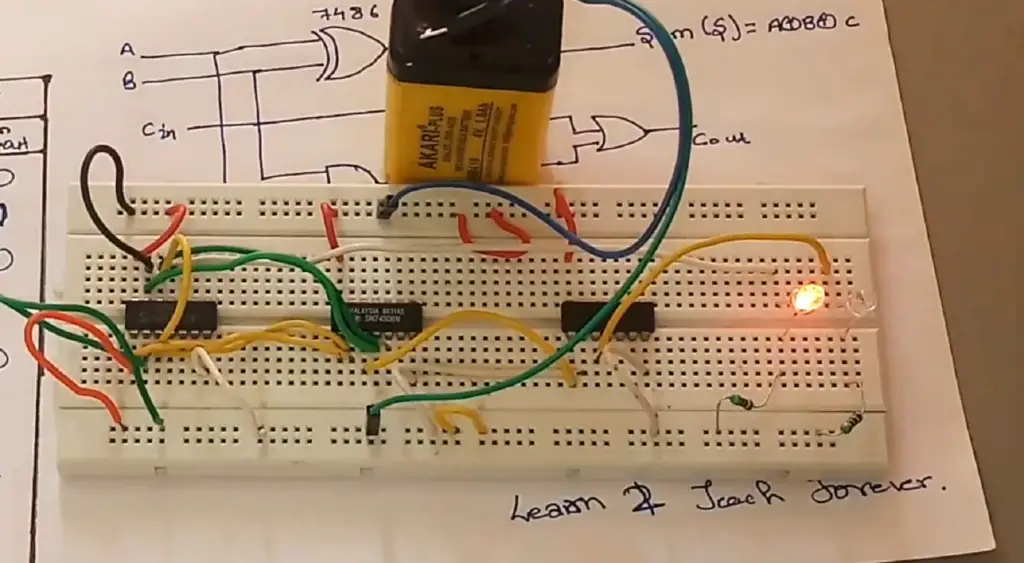The full adder circuit can be implemented using different logic gates, such as AND, OR, XOR, and NAND gates. The choice of gates used depends on the specific design requirements and the intended application. The design of a full adder circuit can also be optimized for minimum propagation delay, reduced power consumption, and minimized area.

### Flexibility

Full Adder circuits are highly flexible, as they can be used to add numbers of different bit lengths by cascading multiple Full Adders. This cascading effect allows for the implementation of complex arithmetic functions. Full Adders can also be used for subtraction, and Boolean logic operations.

### Carry information

One of the significant advantages of using a Full Adder is its ability to generate carry information from the addition of multiple bits. This carry information is essential for implementing arithmetic functions such as adding large numbers and performing operations on numbers that have more significant bits than the circuit’s architecture.

### Speed

Full Adder circuits are among the fastest digital circuits available, which makes them highly desirable in applications that require high-speed arithmetic functions. As Full Adders are used in arithmetic units in microprocessors, their speed is crucial in enhancing the microprocessor’s overall performance.

Therefore, Full Adders are employed in the arithmetic circuits of microprocessors, digital signal processors, and other digital systems that require high-speed arithmetic capabilities.### Complexity

Full adders can be relatively complex compared to simpler logic circuits like AND or OR gates. A basic full adder requires multiple logic gates, such as XOR, AND, and OR gates, to perform addition. These gates introduce additional circuitry, increasing the complexity of the design. As a result, the implementation of full adders can be more demanding in terms of the number of components, space requirements, and power consumption. Complex circuits may also pose challenges during fabrication, testing, and troubleshooting processes, which can increase costs and development time.

### Propagation Delay

Propagation delay refers to the time it takes for an input change to affect the corresponding output of a circuit. In the case of a full adder, the carry signal needs to propagate through each stage to calculate the correct sum and carry-out values. This carry propagation introduces a delay in the circuit, and the time required to obtain the correct output increases with the number of bits being added. This delay can be problematic in applications where speed is crucial, such as high-frequency digital systems or real-time data processing. The longer the propagation delay, the slower the overall operation of the system.

To mitigate the propagation delay issue, various techniques have been developed, such as using faster technologies, optimizing circuit layouts, or employing specialized adder designs like carry-lookahead or carry-select adders. These techniques aim to minimize the impact of propagation delay and enhance the overall performance of the adder circuit.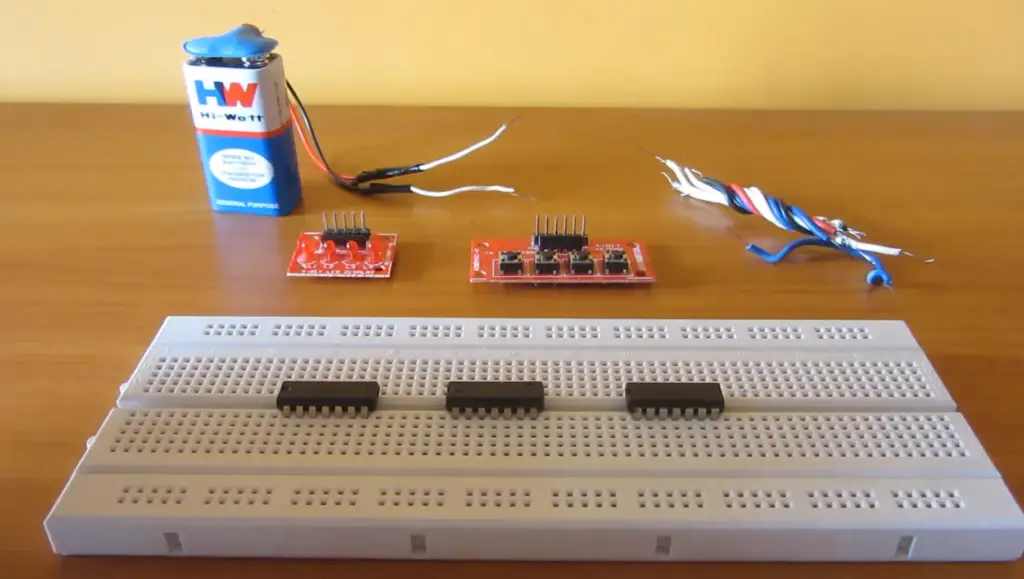## Application of Full Adder in Digital Logic:

### Arithmetic circuits

As a binary adders, Full Adders are extensively used in arithmetic circuits, which are essential in most digital systems. These circuits are used in the implementation of various arithmetic functions such as addition, subtraction, multiplication, and division. Full Adders are used to perform binary addition, which is one of the fundamental arithmetic operations in digital systems. By cascading multiple Full Adders, complex arithmetic operations can be implemented. In addition, Full Adders can also be used to implement algebraic functions and Boolean logic operations.

### Data handling

Full Adders are also used in data handling circuits, which are used to manipulate digital data. These circuits are essential in serial interfaces, where data is transmitted one bit at a time. Full Adders are used in data serialization and de-serialization circuits, which convert serial data into parallel data and vice versa. These circuits are used in devices such as USB, Ethernet, and other communication protocols .

### Counters

Full Adders are also used in counter circuits, which are used to count the number of pulses that occur over time. Counters are used in digital clock circuits, frequency dividers, and in the creation of sequential logic circuits. Full Adders are used to implement binary up/down counters, which count in both directions (up and down). The outputs of Full Adders are used to create multiple stages of counters that can count very large numbers.

### Multiplexers and demultiplexers

Multiplexers and demultiplexers are essential in digital systems for the routing of data from one location to another. These circuits use Full Adders to implement input/output expansion and the selection of different sources for data transfer. Multiplexers are used to select one of several inputs, while demultiplexers are used to route a single input to one of several outputs.

### Memory tending

Full Adders can be used in memory tending circuits, which are used to read/write data from/to memory modules. Memory tending circuits are used in Random Access Memory (RAM) and Read Only Memory (ROM) modules and in caching systems.

### ALUs

Full Adders are also used in arithmetic logic units (ALUs), which are critical components in central processing units (CPUs). ALUs are used to perform arithmetic and Boolean logic operations on operands stored in registers. Full Adders are used in the addition/subtraction unit of ALUs, which performs binary addition and subtraction. Full Adders are also used in the carry-lookahead unit (CLA) of ALUs, which is used to eliminate carry propagation delays in large arithmetic operations.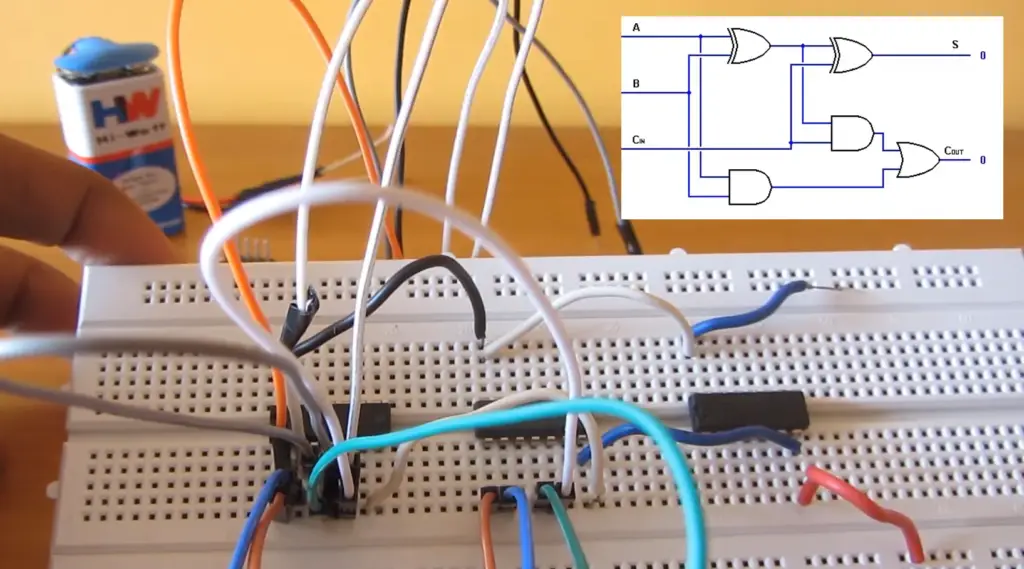The Full Adder circuit is a combinational logic circuit that performs binary addition on three inputs (two single-bit inputs and a carry input) to produce two outputs (a single-bit sum output and a carry output). The construction of a Full Adder circuit involves the use of basic logic gates such as AND gates, OR gates, and XOR gates.

A Full Adder circuit can be implemented using two Half Adders and an OR gate. A Half Adder is a combinational circuit that performs binary addition on two input bits to produce two outputs: a single-bit sum and a carry bit. By combining two Half Adders with an OR gate, we can implement a Full Adder circuit.

The construction of the Full Adder circuit using Half Adders and an OR gate is as follows:

1. Connect the two single-bit input bits to the input of two Half Adders;
2. Connect the carry output of the first Half Adder to the carry input of the second Half Adder;
3. Connect the output sum bits of both Half Adders to the inputs of an OR gate;
4. The output of the OR gate produces the two outputs of the Full Adder: the carry output and the sum output;

Alternatively, the Full Adder circuit can be implemented using XOR (exclusive OR) and AND gates. The use of XOR and AND gates simplifies the circuit design and reduces the number of gates required .

The construction of the Full Adder circuit using XOR and AND gates is as follows:

1. Connect the two single-bit input bits to the input of two XOR gates;
2. Connect the output of one of the XOR gates to the input of an AND gate with the other input being the carry input;
3. Connect the output of the other XOR gate to the input of another AND gate with the other input being the output of the previous AND gate;
4. The output of the AND gate produces the carry output of the Full Adder;
5. The output of the other XOR gate produces the sum output of the Full Adder;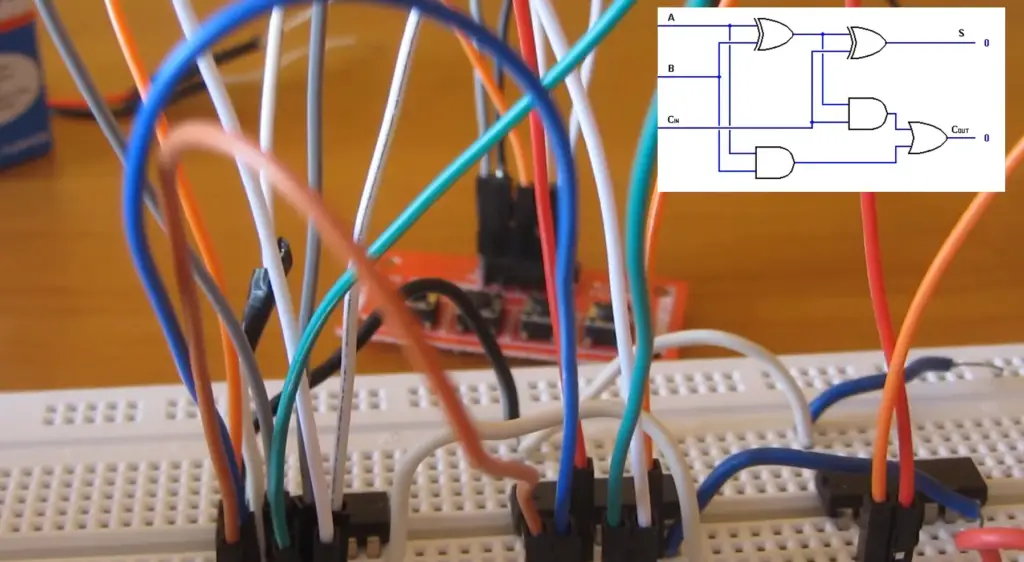Cascading adder circuits refer to a technique used to create adders of higher bit widths by cascading multiple adders together. In this technique, the carry-out of one adder is connected to the carry-in of the next adder, allowing for the addition of multiple numbers with more bits.

For example, to create a 4-bit adder, four 1-bit full adders can be cascaded together. The output of the rightmost adder provides the sum of the input bits, and the carry-out from this adder provides the overflow bit. The circuit can be scaled up to handle larger bit widths by cascading more adders together.

Cascading adder circuits are widely used in digital electronics, including in the design of microprocessors and other computing systems. The technique allows for the creation of powerful and flexible circuits that can perform complex arithmetic operations on large numbers.

## Implementation of Full Adder Using:

A Half Adder is a basic digital logic circuit that adds two binary numbers of one bit each.

It has two inputs, A and B, and two outputs – the sum (S), which is the least significant bit (LSB), and a carry (C), which is the most significant bit (MSB). Two Half Adders can be connected to implement a Full Adder.

To implement a Full Adder using Half Adders, the first Half Adder calculates the sum of the two least significant bits A and B, then the second Half Adder calculates the sum of C (the carry input) and the sum output of the first Half Adder. The output of the second Half Adder is the final S bit of the sum, while its carry output becomes the carry input to the next Full Adder.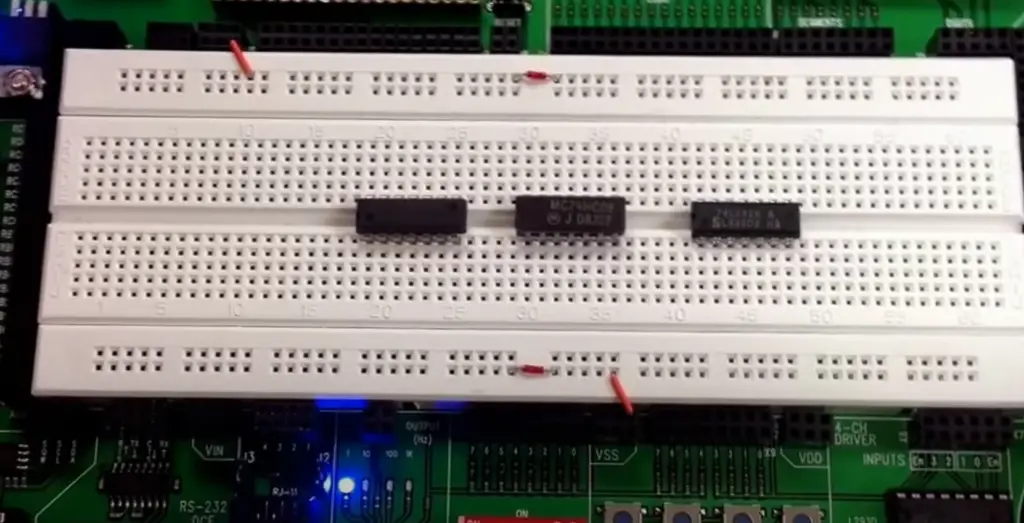### NAND Gates

A NAND gate is a digital logic circuit that implements the logical operation NOT-AND. As its name implies, it is a combination of a NOT gate and an AND gate. NAND gates can be used to implement a Full Adder circuit, with multiple NAND gates connected in different ways.

The Full Adder can be implemented using two 3-input NAND gates. The first NAND gate receives A, B, and the carry input, C, as inputs, and its output is connected to the input of the second NAND gate, which receives A, B, and the output of the first NAND gate as inputs. The output of the second NAND gate is the sum output, S, while its carry output goes to the next Full Adder.

### NOR Gates

A NOR gate is a digital logic circuit that implements the logical operation NOT-OR. NOR gates can also be used to implement a Full Adder circuit.

The Full Adder can be implemented using two 3-input NOR gates. The first NOR gate receives A, B, and the complement of the carry input, C, as inputs, and the second NOR gate receives A, B, and the output of the first NOR gate. The output of the second NOR gate is the sum output, S, while its carry output goes to the next Full Adder. It is important to note that the specific implementation may vary depending on the available components and design requirements.

Using NOR gates has some benefits over other implementations as it has higher noise resistance and simplicity due to fewer logic gates required. NOR gate implementation also has the advantage of being able to implement a Full Subtractor circuit using the same components.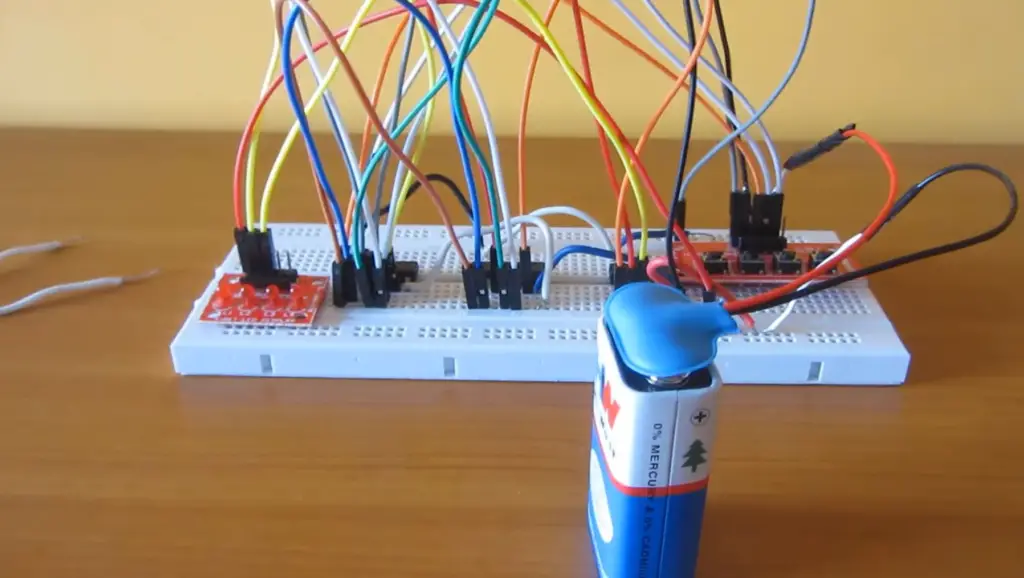## Practical Demonstration of Full Adder Circuit

To practically demonstrate a Full Adder circuit, you would need a breadboard, logic gates, resistors, LED lights, and wires. The Full Adder circuit can be implemented using different logic gates, but one common way is to use two half adders to add the three bits and generate the two outputs.

Here are the steps to demonstrate the Full Adder circuit using two half adders:

1. Connect two inputs (A and B) to the two inputs of the first half adder circuit. Connect the carry output of the first half adder to the carry input of the second half adder;
2. Connect the sum output of the first half adder to one input of the second half adder, and the third input (C-in) to the other input of the second half adder;
3. Connect the sum output of the second half adder to an LED light, which represents the sum output of the Full Adder circuit;
4. Connect the carry output of the second half adder to another LED light, which represents the carry output of the Full Adder circuit;
5. Connect power (VCC) and ground (GND) to the circuit;
6. Test the Full Adder circuit by providing different combinations of input values and observing the corresponding output values on the LED lights;

This is just one example of how to practically demonstrate a Full Adder circuit, and there are numerous other ways to implement and test the circuit. It is important to note that the specific implementation may vary depending on the available components and design requirements.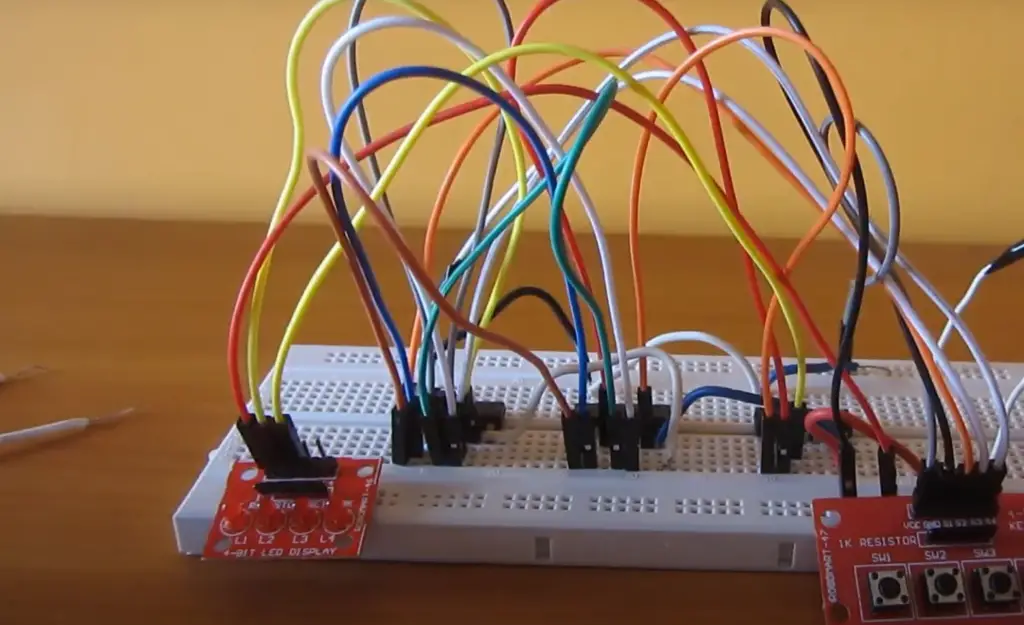## FAQ

### 1. What are the components required for a full adder?

A full adder is a digital circuit that performs addition of three binary inputs: A, B, and a carry-in (Cin). The components required for a full adder are:

• Two XOR gates: These gates take two inputs and produce an output that is true (logic 1) when the inputs differ;
• Two AND gates: These gates take two inputs and produce an output that is true (logic 1) only when both inputs are true;
• One OR gate: This gate takes two inputs and produces an output that is true (logic 1) when at least one of the inputs is true;
• One carry-out (Cout): This is the output that represents the carry generated by the addition operation ;

### 2. What is the principle of a full adder?

The principle of a full adder is based on binary addition. It takes three inputs: A, B, and Cin. The inputs A and B represent the bits to be added, and Cin represents the carry-in from the previous stage. The full adder generates two outputs: the sum (S) and the carry-out (Cout). The sum output represents the least significant bit of the addition result, while the carry-out represents the most significant bit. The full adder considers the inputs and the carry-in to produce the sum and determine whether a carry-out is generated.

### 3. What are the types of full adder circuits?

There are mainly two types of full adder circuits:

• Ripple Carry Adder: In this type, multiple full adders are cascaded, and the carry-out of each adder is connected to the carry-in of the next adder. The carry ripples through the adders, hence the name “ripple carry adder”;
• Carry Lookahead Adder: This type of adder uses additional logic to generate carry signals in parallel for all the bits being added. It reduces the propagation delay associated with ripple carry adders, making it faster ;

### 4. Why do we use a full adder?

We use a full adder to perform addition operations in digital systems. It is a fundamental building block for arithmetic circuits and is widely used in microprocessors, calculators, and other digital devices. The full adder allows us to add binary numbers and handle carry bits, enabling the addition of numbers larger than a single bit.

A single full adder can add two bits, along with a carry-in. The inputs A and B represent the two bits to be added, and the carry-in (Cin) represents the carry-bit from the previous stage. The output sum (S) represents the least significant bit of the result, and the carry-out (Cout) represents the most significant bit.

### 6. How many full adders are required to construct?

The number of full adders required to construct an arithmetic circuit depends on the number of bits being added. For an n-bit addition, where n represents the number of bits in the binary numbers being added, you would need n full adders. Each full adder handles one bit of the addition operation.

### 7. How many gates are used in a full adder?

A full adder circuit requires a total of 9 gates. Here’s the breakdown:

• Two XOR gates: These gates are used to calculate the sum (S) output. One XOR gate is used for inputs A and B, and the other XOR gate is used for the output of the first XOR gate and the carry-in (Cin)’
• Four AND gates: These gates are used for various combinations of inputs to generate the carry-out (Cout) and the carry signal for the next stage. Two AND gates are used for the inputs A and B, one AND gate is used for the output of the first XOR gate and the carry-in (Cin), and one AND gate is used for the inputs A and B with an inverted carry-in (Cin)’
• One OR gate: This gate is used to combine the outputs of the two AND gates connected to the inputs A and B. The OR gate generates the carry-out (Cout) ;

### 8. What is the maximum frequency of a full adder?

The maximum frequency of a full adder circuit depends on various factors, including the technology used, the complexity of the circuit, and the characteristics of the components. In general, modern digital circuits can operate at high frequencies, ranging from several megahertz to several gigahertz. However, the exact maximum frequency of a full adder can vary significantly based on the specific implementation and design considerations.

### 9. How many types of adders are there?

There are several types of adders used in digital systems, including:

• Half Adder: Adds two single binary digits and generates the sum and carry outputs;
• Full Adder: Adds three single binary digits (two inputs and a carry-in) and produces the sum and carry outputs;
• Carry Lookahead Adder: Uses additional logic to generate carry signals in parallel, reducing the propagation delay;
• Carry Select Adder: Provides faster addition by using multiplexers to select the carry generated by either a fast adder or a slow adder, depending on the input operands;

These are some of the commonly used types of adders, but there are other variations and specialized adders as well.

### 10. How do you build a full adder?

A full adder can be built using basic logic gates, such as XOR, AND, and OR gates. Here’s a step-by-step procedure to construct a full adder:

1. Connect the inputs A and B to two XOR gates. The outputs of these XOR gates represent the XORed results of A and B;
2. Connect the XOR gate outputs to two separate inputs of AND gates;
3. Connect the input Cin to an additional input of one of the AND gates;
4. Connect the outputs of the two AND gates to the inputs of an OR gate;
5. The output of the OR gate represents the carry-out (Cout), and the XOR gate outputs represent the sum (S) output;

### 11. How do you connect a full adder to a circuit?

To connect a full adder to a circuit, you would typically connect the inputs (A, B, and Cin) of the full adder to the corresponding signals in the circuit. The sum (S) output and carry-out (Cout) of the full adder can be connected to the desired destinations or used as inputs to other components in the circuit, depending on the specific application.

### 12. What is a 4-bit full adder?

A 4-bit full adder is a circuit that can perform addition on 4-bit binary numbers.

It consists of four full adders connected together to handle each bit of the addition operation. The carry-out (Cout) of each full adder is connected to the carry-in (Cin) of the next full adder, allowing the addition to propagating through all four bits.

### 13. Which is the fastest full adder?

The fastest full adder design can vary depending on the specific technology and design considerations.

However, one commonly cited fast full adder design is the Kogge-Stone adder. The Kogge-Stone adder is a parallel prefix adder that utilizes a tree structure to compute the carry signals in parallel. This design reduces the critical path delay and enables high-speed addition. Other fast adder designs include the Brent-Kung adder and the Han-Carlson adder, which also employ parallel prefix structures to optimize speed.

### 14. Is 7483 a full adder?

No, the 7483 is not a full adder. The 7483 is a 4-bit binary full-adder IC. It combines four individual full adders in a single integrated circuit package, allowing it to perform addition on 4-bit binary numbers. Each full adder within the 7483 IC has three inputs (A, B, and Cin) and two outputs (Sum and Carry). The 7483 IC is commonly used in digital systems for multi-bit addition.

### 15. Can a full adder have 4 inputs?

No, a standard full adder does not have 4 inputs. A full adder is designed to add two binary digits (A and B) along with a carry-in (Cin). Therefore, it has three inputs: A, B, and Cin. However, it is possible to extend the functionality of a full adder by adding an additional input if needed.

For example, by introducing an extra input, you could create a full adder with an additional input for a carry-in from a previous stage, resulting in a 4-input full adder.

### 16. What is the minimum number of gates to design a full adder circuit?

The minimum number of gates required to design a full adder circuit is 5 gates. This includes 2 XOR gates, 2 AND gates, and 1 OR gate. This minimal configuration allows for the implementation of a basic full adder functionality.

### 17. How many transistors does a full adder have?

The number of transistors in a full adder circuit depends on the specific technology and design choices. In a CMOS (Complementary Metal-Oxide-Semiconductor) implementation, a basic full adder typically requires around 28 to 32 transistors .

### 18. What is the maximum size of an adder?

The maximum size of an adder refers to the number of bits it can handle. Adders can be designed to add binary numbers of various sizes. The size of an adder is typically indicated by the number of bits it can accommodate.

For example, a 4-bit adder can add two 4-bit binary numbers together, while an 8-bit adder can add two 8-bit binary numbers together. The maximum size of an adder depends on the specific requirements of the digital system and the available resources.

### 19. How many full adders are required for an 8-bit adder?

To construct an 8-bit adder, you would need eight full adders. Each full adder handles one bit of the addition operation. By connecting the carry-out (Cout) of each full adder to the carry-in (Cin) of the next full adder, you can perform addition across multiple bits.

### 20. Does a full adder have memory?

No, a full adder does not have memory. A combinational logic circuit performs addition on binary inputs and generates outputs based on the inputs and the carry-in signal. A full adder does not store or retain any information once the inputs and carry-in change.

### 21. Why do we need a full adder or subtractor?

A full adder or subtractor is needed to perform arithmetic operations in digital systems. A full adder is used to add binary numbers, while a subtractor is used to perform subtraction. These operations are fundamental in various applications such as calculators, microprocessors, digital signal processing, and other arithmetic-intensive tasks.